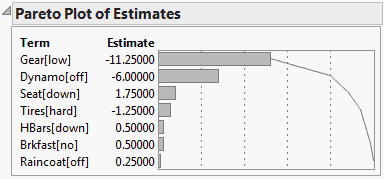Publication date: 04/12/2021

## Pareto Plot Report

The Pareto Plot report presents a Pareto chart of the absolute values of the estimates. Figure 3.47 shows a Pareto Plot report for the Bicycle.jmp sample data table.

If the original estimates have equal variances and are not correlated, the original estimates are plotted.

If the original estimates have unequal variances and are not correlated, the t ratios are plotted.

If the original estimate have unequal variances and are correlated, the Orthog Coded estimates are plotted.

The cumulative sum line in the plot sums the absolute values of the estimates. Keep in mind that the orthogonal estimates depend on the order of entry of terms into the model.

Note: The estimates that appear in the plot are standardized to have equal variances and are transformed to be orthogonal. You have the option of undoing these transformations. See Transformations.

Figure 3.47 Pareto PlotWant more information? Have questions? Get answers in the JMP User Community (community.jmp.com).